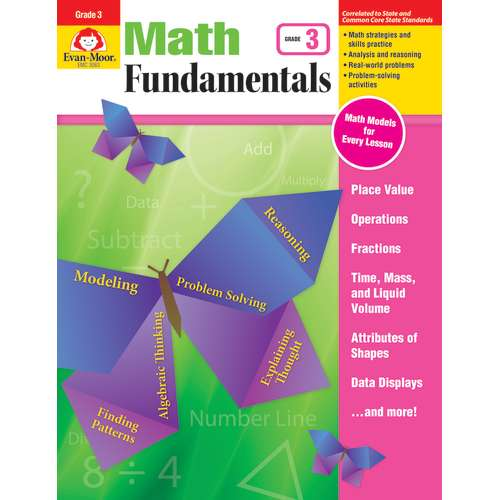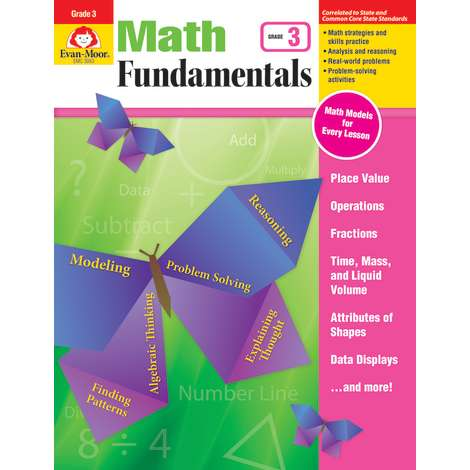•Product Number: EMC3083
\$19.99
Comprehensive but not complicated! Math Fundamentals helps your third grade students navigate the new math. Math Models and “...
Quantity

Description

Comprehensive but not complicated! Math Fundamentals helps your third grade students navigate the new math. Math Models and “think” questions, plenty of skill practice, and real-world problems guide students in thinking through, analyzing, and solving problems.

To help you target instruction, each unit clearly lists the standards information, mathematical practices, and skills covered.

Within a unit, math lessons are presented simply. Every math lesson includes:
• A Math Models reference page that shows students strategies for solving problems. This is helpful for parents and students transitioning to new math.
• Skill practice pages that progress in difficulty.
• A culminating problem-solving activity that leads students through the process of solving a real-life problem.
• Solve problems with multiplication and division
• Use properties of multiplication and division
• Multiply and divide within 100
• Solve problems and identify patterns
• Perform multidigit arithmetic
• Understand fractions
• Measure time, liquid volumes, and masses
• Represent and interpret data
• Measure area
• Measure perimeter
• Analyze shapes and their attributes
Designed to support new math programs, Math Fundamentals is the perfect easy-to-use supplement to your Common Core math or current math curriculum.

Better together! Use Math Fundamentals with Daily Math Practice for complementary skill practice that ensures your students master grade-level math.

This resource contains teacher support pages, reproducible student pages, and an answer key.

EMC3083